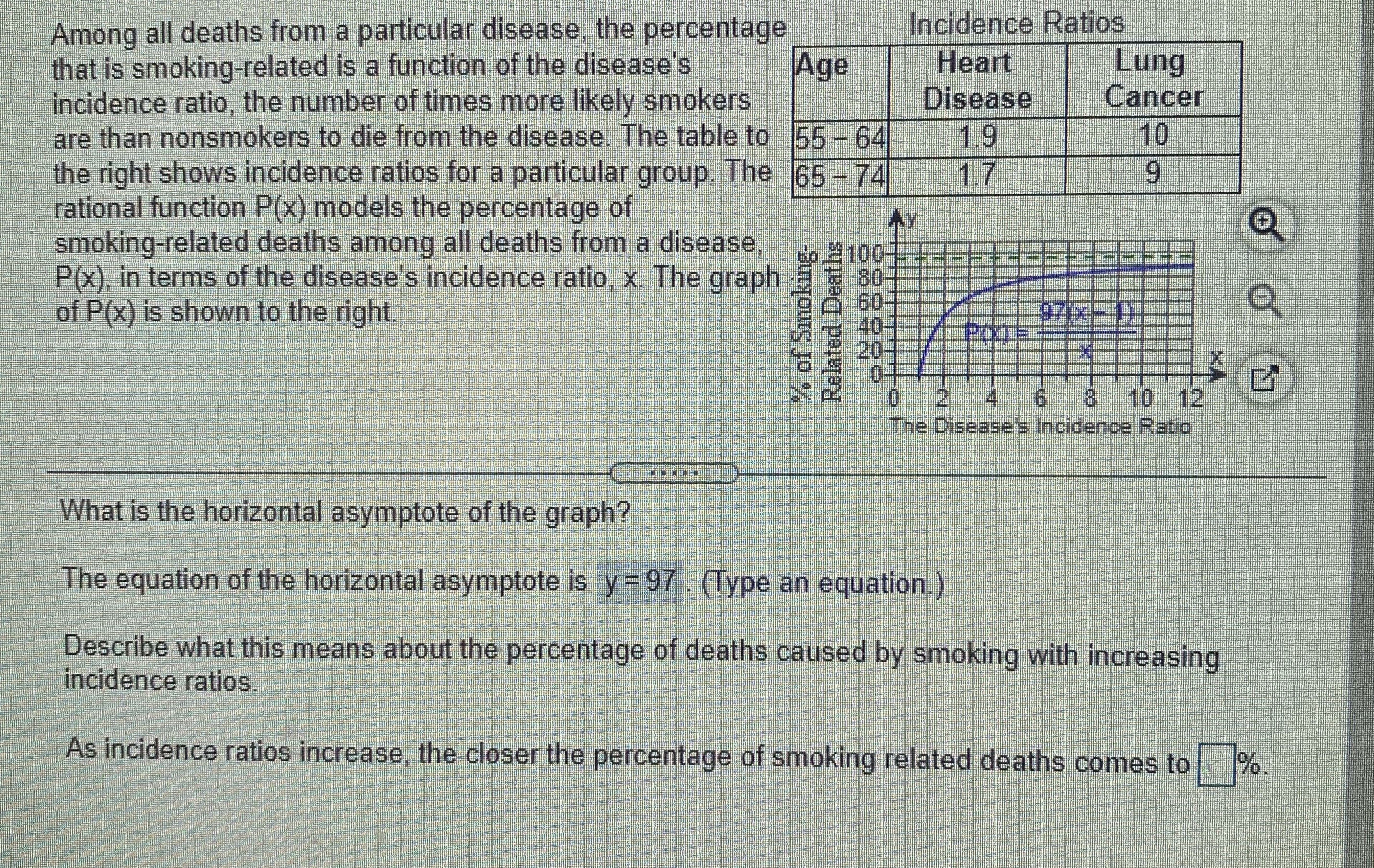### Still have math questions?

Algebra
QuestionAmong all deaths from a particular disease, the percentage that is smoking-related is a function of the disease's incidence ratio, the number of times more likely smokers are than nonsmokers to die from the disease. The table to the right shows incidence ratios for a particular group. The rational function $$P ( x )$$ models the percentage of smoking-related deaths among all deaths from a disease,

$$P ( x )$$ , in terms of the disease's incidence ratio, $$x$$ . The graph of $$P ( x )$$ is shown to the right.

What is the horizontal asymptote of the graph? The equation of the horizontal asymptote is $$y = 97$$ . (Type an equation.) Describe what this means about the percentage of deaths caused by smoking with increasing incidence ratios. As incidence ratios increase, the closer the percentage of smoking related deaths comes to $$\square$$ \%.